We know that that the rhombic dodecahedron is a polyhedron related with how bees build honeycombs. The bottom of a cell (the keel as Kepler called it) is made by three rhombuses. And Kepler knew that with twelve of these rhombi we can build a polyhedron called rhombic dodecahedron.Humankind has always been fascinated by how bees build their honeycombs. Kepler related honeycombs with a polyhedron called Rhombic Dodecahedron.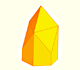We want to close a hexagonal prism as bees do, using three rhombi. Then, which is the shape of these three rhombi that closes the prism with the minimum surface area?.

In this page we are going to take another point of view.

One way to build new polyhedra is start with one known polyhedra and add pyramids in each of their faces.

This is a very well known technique. For example, Luca Pacioli studied several of these polyhedra in his book "De Divine Proportione" (around 1500).

Pacioli wrote about the "Augmented cube" and he called it "exacedron elevatus" (Spanish translation):

"[El hexaedro elevado sólido o hueco] está compuesto por seis pirámides laterales cuadriláteras exteriores, que se ofrecen a nuestra vista según la situación del cuerpo. En cuanto al cubo interior sobre el que se apoyan dichas pirámides, y que sólo puede ser imaginado por el intelecto pues se oculta a nuestra vista por la superposición de dichas pirámides, sus seis superficies cuadradas son bases de las mencionadas seis pirámides, que son todas de la misma altura, y se ocultan a la vista y circundan a dicho cubo."
('La divina proporción' de Luca Pacioli, page 92, Spanish translation by Juan Calatrava, Editorial Akal, 4th edition, 2008)

These drawings were made by Leonardo da Vinci: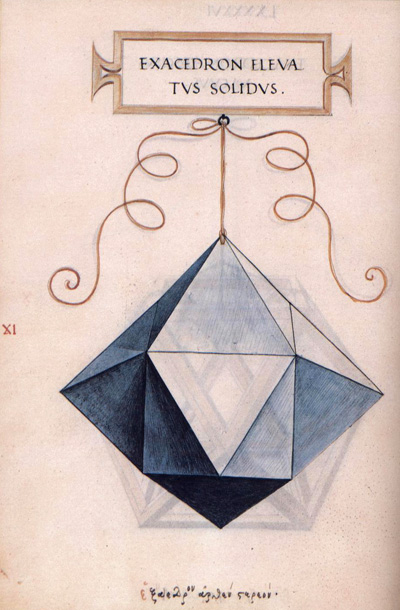Playing with transprency we can see the interior cube:You can separate the pyramids:One interesting fact is that you can make that these twenty four triangular faces become twelve rhombuses and then you get a new polyhedron called Rhombic Docecahedron: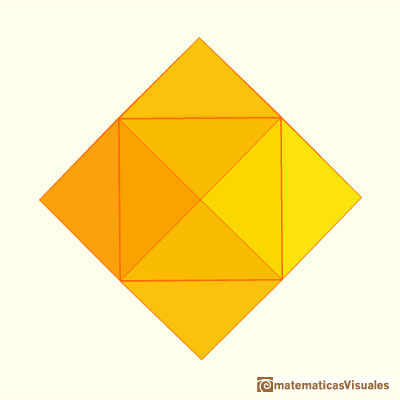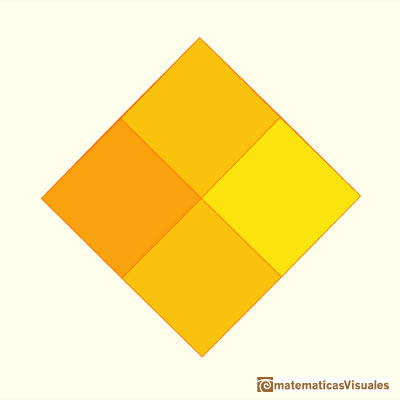We are going to calculate the diagonal D of one of these rhombuses: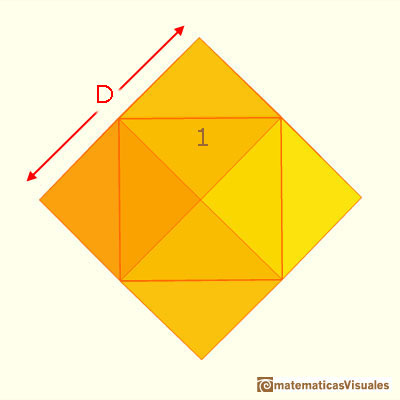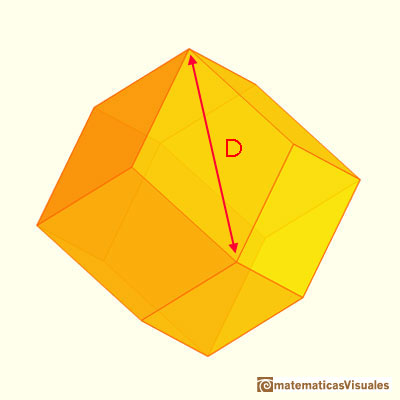These are the same rhombuses that we found studying honeycombs.We want to close a hexagonal prism as bees do, using three rhombi. Then, which is the shape of these three rhombi that closes the prism with the minimum surface area?.

The length of the edge of this rhombic dodecahedron is:These rhombuses are related with the proportions of the standard size of paper DinA.There is a standarization of the size of the paper that is called DIN A. Successive paper sizes in the series A1, A2, A3, A4, and so forth, are defined by halving the preceding paper size along the larger dimension.

You can use trigonometry to calculate the angles of one rhombi: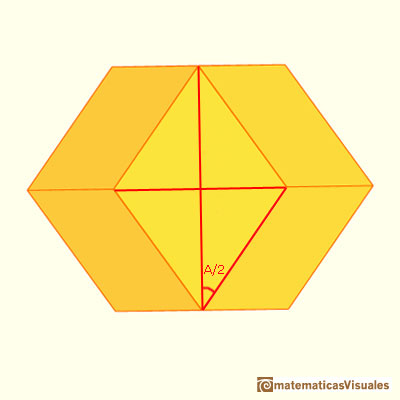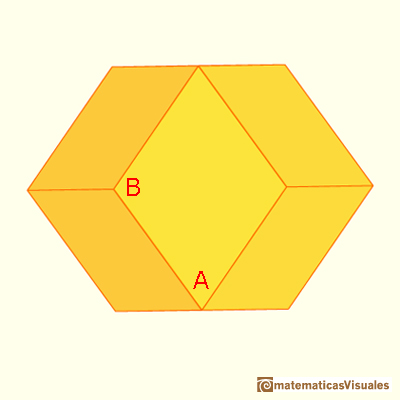Johannes Kepler was the first mathematician to write about the rhombic dodecahedron. For example, this is a drawing in his book "Harmonices Mundi":Some garnet cristals have this shape:REFERENCES

Johannes Kepler - The Six Cornered Snowflake: a New Year's gif - Paul Dry Books, Philadelphia, Pennsylvania, 2010. English translation of Kepler's book 'De Nive Sexangula'. With notes by Owen Gingerich and Guillermo Bleichmar and illustrations by the spanish mathematician Capi Corrales Rodrigáñez.
D'Arcy Thompson - On Growth And Form - Cambridge University Press, 1942.
Hugo Steinhaus - Mathematical Snapshots - Oxford University Press - Third Edition.
Magnus Wenninger - 'Polyhedron Models', Cambridge University Press.
Peter R. Cromwell - 'Polyhedra', Cambridge University Press, 1999.
H.Martin Cundy and A.P. Rollet, 'Mathematical Models', Oxford University Press, Second Edition, 1961.
W.W. Rouse Ball and H.S.M. Coxeter - 'Matematical Recreations & Essays', The MacMillan Company, 1947.Starting with a Rhombicubotahedron we can add pyramids over each face. The we get a beautiful polyhedron that it is like a star.Leonardo da Vinci made several drawings of polyhedra for Luca Pacioli's book 'De divina proportione'. Here we can see an adaptation of the augmented rhombicuboctahedron.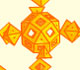We can see the interior of the augmented rhombicuboctahedron. Luca Pacioli wrote that you 'can see the interior only with your imagination'.Humankind has always been fascinated by how bees build their honeycombs. Kepler related honeycombs with a polyhedron called Rhombic Dodecahedron.A chain of six pyramids can be turned inwards to form a cube or turned outwards, placed over another cube to form the rhombic dodecahedron.Material for a session about polyhedra (Zaragoza, 9th May 2014). Simple techniques to build polyhedra like the tetrahedron, octahedron, the cuboctahedron and the rhombic dodecahedron. We can build a box that is a rhombic dodecahedron.There is a standarization of the size of the paper that is called DIN A. Successive paper sizes in the series A1, A2, A3, A4, and so forth, are defined by halving the preceding paper size along the larger dimension.Leonardo da Vinci made several drawings of polyhedra for Luca Pacioli's book 'De divina proportione'. Here we can see an adaptation of the truncated octahedron.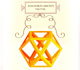Leonardo da Vinci made several drawings of polyhedra for Luca Pacioli's book 'De divina proportione'. Here we can see an adaptation of the cuboctahedron.A cuboctahedron is an Archimedean solid. It can be seen as made by cutting off the corners of a cube.A cuboctahedron is an Archimedean solid. It can be seen as made by cutting off the corners of an octahedron.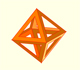The volume of an octahedron is four times the volume of a tetrahedron. It is easy to calculate and then we can get the volume of a tetrahedron.The truncated octahedron is an Archimedean solid. It has 8 regular hexagonal faces and 6 square faces. Its volume can be calculated knowing the volume of an octahedron.These polyhedra pack together to fill space, forming a 3 dimensional space tessellation or tilling.You can chamfer a cube and then you get a polyhedron similar (but not equal) to a truncated octahedron. You can get also a rhombic dodecahedron.A Cube can be inscribed in a Dodecahedron. A Dodecahedron can be seen as a cube with six 'roofs'. You can fold a dodecahedron into a cube.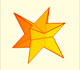If you fold the six roofs of a regular dodecahedron into a cube there is an empty space. This space can be filled with an irregular dodecahedron composed of identical irregular pentagons (a kind of pyritohedron).